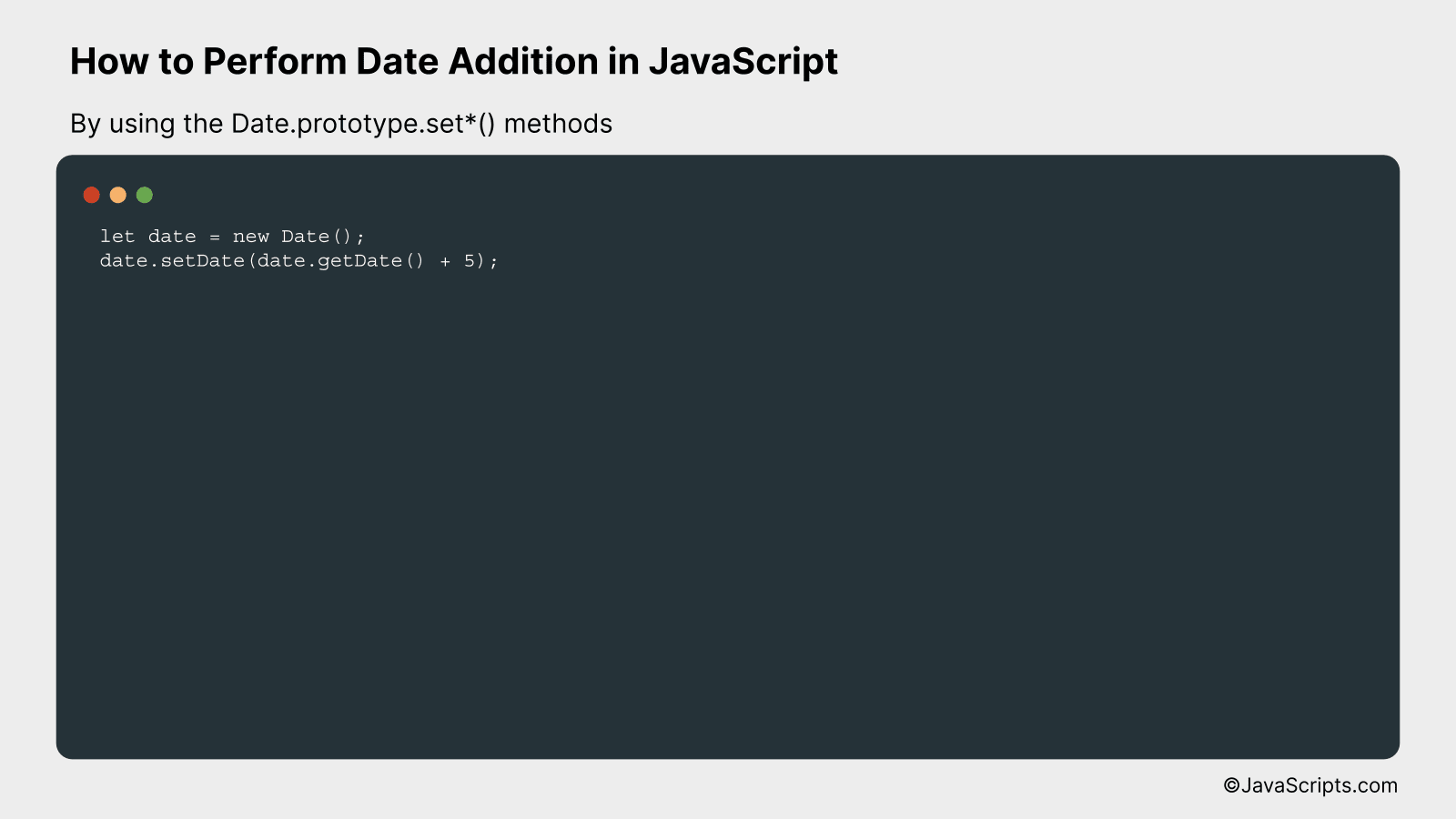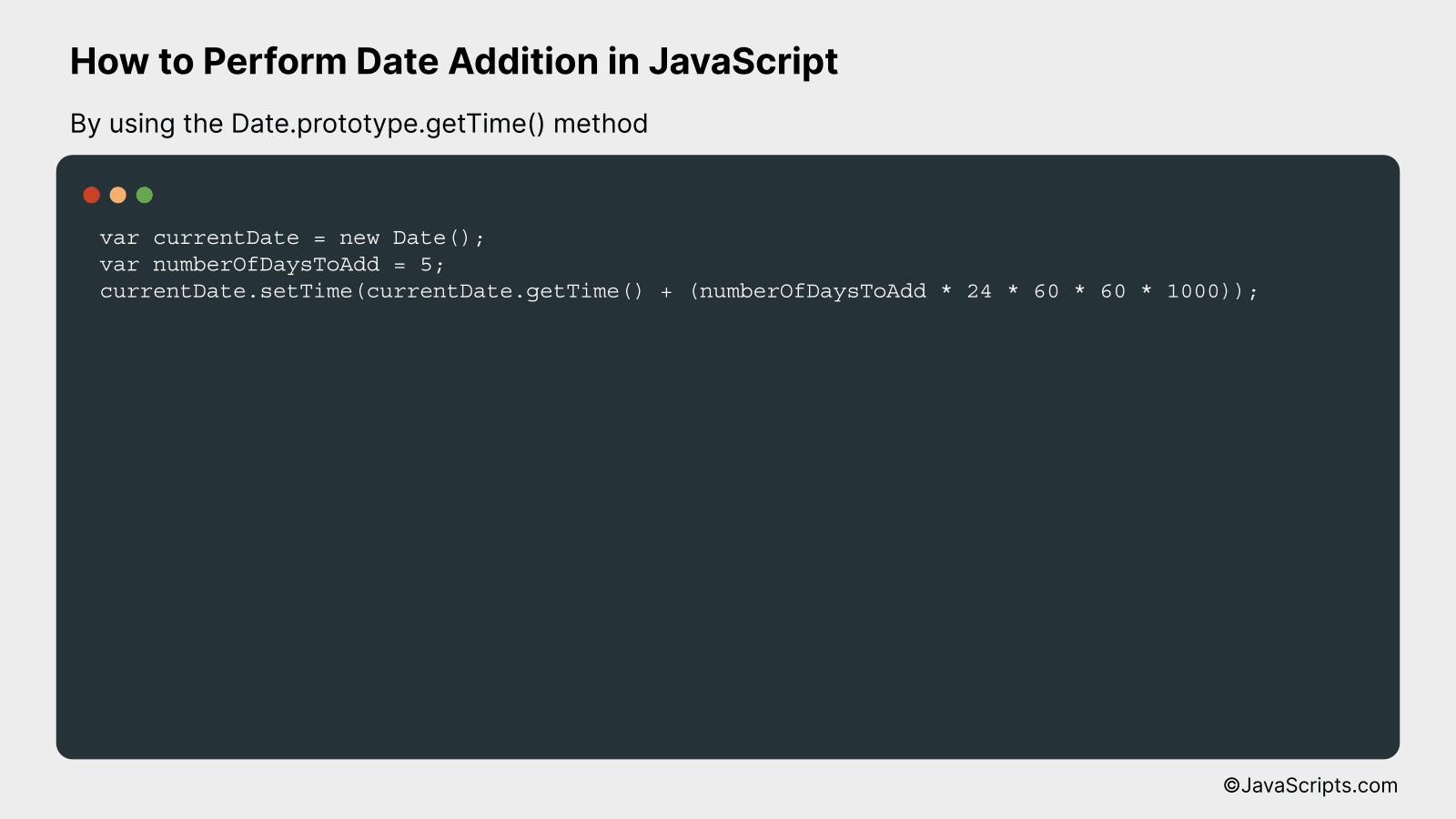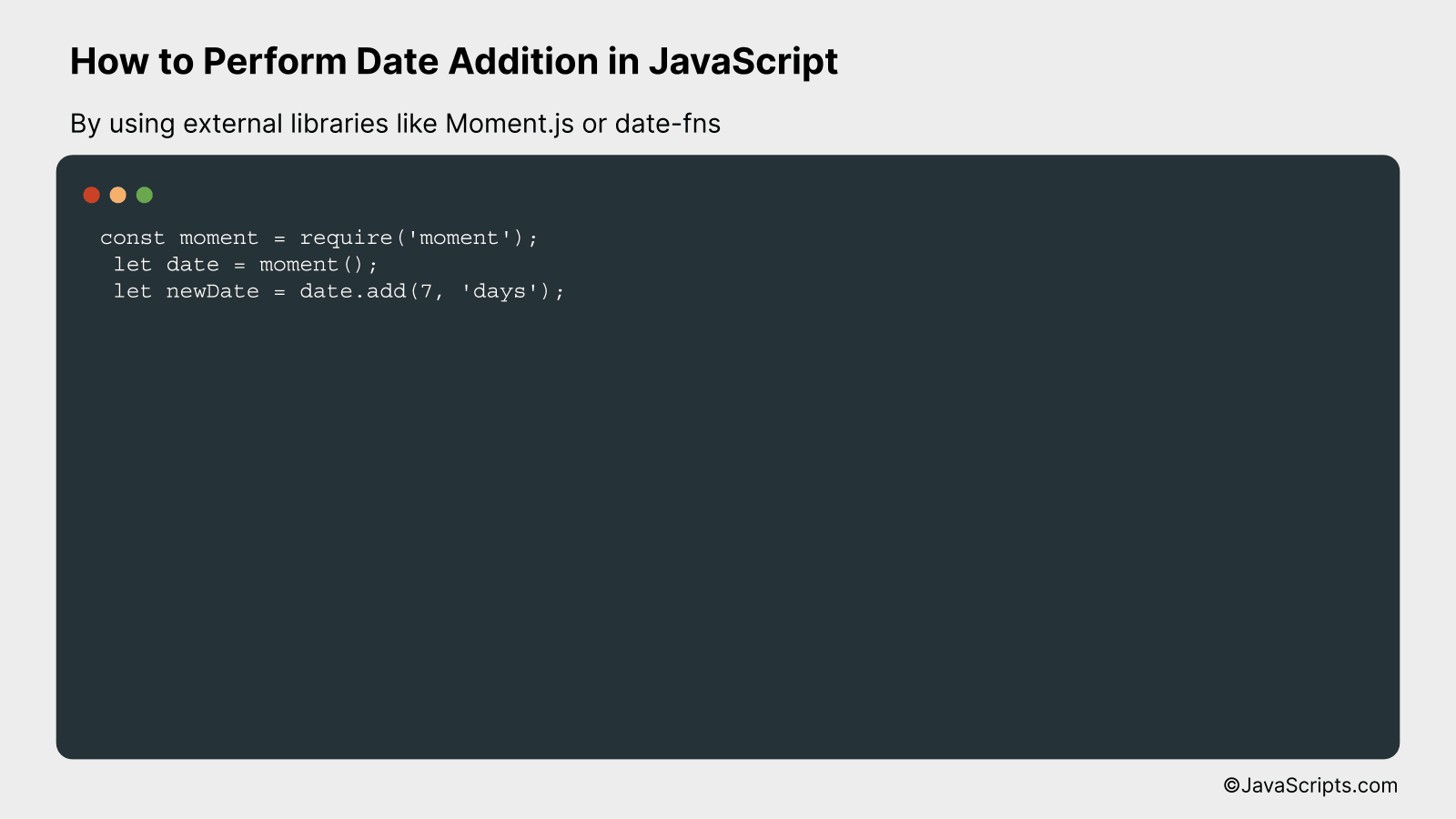# How to Perform Date Addition in JavaScript

Welcome, fellow code enthusiasts! We all know mastering JavaScript opens a whole new world of possibilities, doesn’t it? It’s one of those essential skills that can take your development journey to an incredible height.

So, let’s take a step deeper into this universe today. Specifically, we’re going to explore date addition in JavaScript. Don’t worry if you are a beginner – we’ll break it down to the basics, ensuring you grasp the concept with ease and confidence.

In this post, we will be looking at the following 3 ways to perform date addition in JavaScript:

• By using the Date.prototype.set*() methods
• By using the Date.prototype.getTime() method
• By using external libraries like Moment.js or date-fns

Let’s explore each…

## #1 – By using the Date.prototype.set*() methodsThe JavaScript Date.prototype.set*() methods allow for adding to date parts such as day, month, year, hour, minute, and second by creating a new Date object and then adding to the desired date part. Let’s add 5 days to the current date as an example.

``````
let date = new Date();
date.setDate(date.getDate() + 5);
``````

### How it works

The JavaScript Date object is used to work with dates and times. The setDate() method sets the day of the Date object relative to the beginning of the currently set month. Here, we’re getting the current date using getDate() method and then adding five days to it using setDate() method.

• First, a new Date object is created using `new Date()`. This stores the current date and time.
• Then, the `getDate()` method is used to get the current day as a number (1-31).
• We add 5 to the current day number. This value represents the number of days since the first day of the month.
• Finally, the `setDate()` method is used to update the day of the month for the created Date object.

## #2 – By using the Date.prototype.getTime() methodWe will use the JavaScript Date.prototype.getTime() method to get the current date in milliseconds since the Unix Epoch (January 1, 1970 00:00:00 UTC) and then add the desired number of days converted into milliseconds, to perform date addition. We will then transform the resulting milliseconds back into a Date object. This concept will be easier to understand with the following example:

``````
var currentDate = new Date();
currentDate.setTime(currentDate.getTime() + (numberOfDaysToAdd * 24 * 60 * 60 * 1000));
``````

### How it works

The JavaScript Date.prototype.getTime() method returns the number of milliseconds since the Unix Epoch, which we are using as a basis for our calculations. We multiply the number of days we wish to add by the number of milliseconds in a day (24 hours * 60 minutes * 60 seconds * 1000 milliseconds) to get a time span in milliseconds. This is then added to our current date, also represented in milliseconds. Finally, we set the time of the current date object to this new millisecond count, effectively changing the date.

• Step 1: The current date is retrieved using the ‘new Date()’ function, which is then stored in ‘currentDate’.
• Step 2: The number of days to add is defined in ‘numberOfDaysToAdd’
• Step 3: The getTime() method is called on the ‘currentDate’ object to get the current date and time in milliseconds since the Unix Epoch.
• Step 4: The number of days to add is converted to milliseconds (numberOfDaysToAdd * 24 * 60 * 60 * 1000) and added to the current date and time in milliseconds.
• Step 5: The setTime() method is called on the ‘currentDate’ object to set the date and time by adding the number of milliseconds calculated in step 4. This effectively changes the date of the ‘currentDate’ object.

## #3 – By using external libraries like Moment.js or date-fnsWe will be performing date addition in JavaScript using external libraries like Moment.js or date-fns. These libraries help in handling, formatting, and manipulating dates. We will be adding a specified number of days to a date and will explain its working with an example.

### Example using Moment.js

``````
const moment = require('moment');
let date = moment();
```
```

### How it works

This code snippet uses Moment.js, a widely used JavaScript library for manipulating dates and times. It makes the task of adding days to a date quite simple.

• Step 1: We start by importing the ‘moment’ library.
• Step 2: We create a ‘moment’ object, which by default holds the current date and time.
• Step 3: We use the ‘add’ function from ‘moment’ to add 7 days to the current date. The ‘add’ function takes two parameters: the value to add and the type of unit to add. In this case, we are adding 7 ‘days’.
• Step 4: The new date (current date + 7 days) is stored in the variable ‘newDate’.

### Example using date-fns

``````
const { addDays } = require('date-fns');
let date = new Date();
```
```

### How it works

This code snippet uses date-fns, a modern JavaScript date utility library. It provides a straightforward way to add days to a date.

• Step 1: We start by importing the ‘addDays’ function from the ‘date-fns’ library.
• Step 2: We create a new ‘Date’ object, which by default holds the current date and time.
• Step 3: We use the ‘addDays’ function to add 7 days to the current date. The ‘addDays’ function takes two parameters: the date to change and the amount of days to add.
• Step 4: The new date (current date + 7 days) is stored in the variable ‘newDate’.

Related:

In conclusion, adding dates in JavaScript doesn’t have to be a hurdle. By understanding the JavaScript Date object and functions like ‘setDate’ and ‘getDate’, we can undertake this task effortlessly and accurately.

Remember, practice is key to mastering JavaScript or any programming language. So, keep experimenting with different date scenarios and refine your skills. Happy coding!